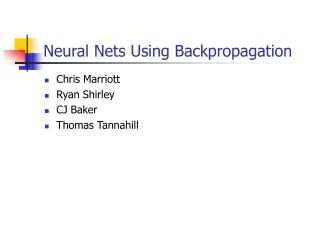# Neural Nets Using Backpropagation - PowerPoint PPT PresentationDownload PresentationNeural Nets Using Backpropagation

Neural Nets Using Backpropagation
Download Presentation## Neural Nets Using Backpropagation

- - - - - - - - - - - - - - - - - - - - - - - - - - - E N D - - - - - - - - - - - - - - - - - - - - - - - - - - -
##### Presentation Transcript

1. Neural Nets Using Backpropagation • Chris Marriott • Ryan Shirley • CJ Baker • Thomas Tannahill

2. Agenda • Review of Neural Nets and Backpropagation • Backpropagation: The Math • Advantages and Disadvantages of Gradient Descent and other algorithms • Enhancements of Gradient Descent • Other ways of minimizing error

3. Review • Approach that developed from an analysis of the human brain • Nodes created as an analog to neurons • Mainly used for classification problems (i.e. character recognition, voice recognition, medical applications, etc.)

4. Output Weightedinputs Activation function = f(S(inputs * weight)) Review • Neurons have weighted inputs, threshold values, activation function, and an output

5. Review 4 Input AND Inputs Threshold = 1.5 Outputs Threshold = 1.5 Inputs Threshold = 1.5 All weights = 1 and all outputs = 1 if active 0 otherwise

6. Review • Output space for AND gate Input 1 (1,1) (0,1) 1.5 = w1*I1 + w2*I2 Input 2 (0,0) (1,0)

7. Review • Output space for XOR gate • Demonstrates need for hidden layer Input 1 (1,1) (0,1) Input 2 (0,0) (1,0)

8. Backpropagation: The Math • General multi-layered neural network Output Layer 0 1 2 3 4 5 6 7 8 9 X9,0 X0,0 X1,0 Hidden Layer 0 1 i Wi,0 W1,0 W0,0 Input Layer 0 1

9. Backpropagation: The Math • Backpropagation • Calculation of hidden layer activation values

10. Backpropagation: The Math • Backpropagation • Calculation of output layer activation values

11. Backpropagation: The Math • Backpropagation • Calculation of error dk = f(Dk) -f(Ok)

12. Backpropagation: The Math • Backpropagation • Gradient Descent objective function • Gradient Descent termination condition

13. Backpropagation: The Math • Backpropagation • Output layer weight recalculation Learning Rate (eg. 0.25) Error at k

14. Backpropagation: The Math • Backpropagation • Hidden Layer weight recalculation

15. Backpropagation Using Gradient Descent • Advantages • Relatively simple implementation • Standard method and generally works well • Disadvantages • Slow and inefficient • Can get stuck in local minima resulting in sub-optimal solutions

16. Local Minima Local Minimum Global Minimum

17. Alternatives To Gradient Descent • Simulated Annealing • Advantages • Can guarantee optimal solution (global minimum) • Disadvantages • May be slower than gradient descent • Much more complicated implementation

18. Alternatives To Gradient Descent • Genetic Algorithms/Evolutionary Strategies • Advantages • Faster than simulated annealing • Less likely to get stuck in local minima • Disadvantages • Slower than gradient descent • Memory intensive for large nets

19. Alternatives To Gradient Descent • Simplex Algorithm • Advantages • Similar to gradient descent but faster • Easy to implement • Disadvantages • Does not guarantee a global minimum

20. Enhancements To Gradient Descent • Momentum • Adds a percentage of the last movement to the current movement

21. Enhancements To Gradient Descent • Momentum • Useful to get over small bumps in the error function • Often finds a minimum in less steps • w(t) = -n*d*y + a*w(t-1) • w is the change in weight • n is the learning rate • d is the error • y is different depending on which layer we are calculating • a is the momentum parameter

22. Enhancements To Gradient Descent • Adaptive Backpropagation Algorithm • It assigns each weight a learning rate • That learning rate is determined by the sign of the gradient of the error function from the last iteration • If the signs are equal it is more likely to be a shallow slope so the learning rate is increased • The signs are more likely to differ on a steep slope so the learning rate is decreased • This will speed up the advancement when on gradual slopes

23. Enhancements To Gradient Descent • Adaptive Backpropagation • Possible Problems: • Since we minimize the error for each weight separately the overall error may increase • Solution: • Calculate the total output error after each adaptation and if it is greater than the previous error reject that adaptation and calculate new learning rates

24. Enhancements To Gradient Descent • SuperSAB(Super Self-Adapting Backpropagation) • Combines the momentum and adaptive methods. • Uses adaptive method and momentum so long as the sign of the gradient does not change • This is an additive effect of both methods resulting in a faster traversal of gradual slopes • When the sign of the gradient does change the momentum will cancel the drastic drop in learning rate • This allows for the function to roll up the other side of the minimum possibly escaping local minima

25. Enhancements To Gradient Descent • SuperSAB • Experiments show that the SuperSAB converges faster than gradient descent • Overall this algorithm is less sensitive (and so is less likely to get caught in local minima)

26. Other Ways To Minimize Error • Varying training data • Cycle through input classes • Randomly select from input classes • Add noise to training data • Randomly change value of input node (with low probability) • Retrain with expected inputs after initial training • E.g. Speech recognition

27. Other Ways To Minimize Error • Adding and removing neurons from layers • Adding neurons speeds up learning but may cause loss in generalization • Removing neurons has the opposite effect

28. Resources • Artifical Neural Networks, Backpropagation, J. Henseler • Artificial Intelligence: A Modern Approach, S. Russell & P. Norvig • 501 notes, J.R. Parker • www.dontveter.com/bpr/bpr.html • www.dse.doc.ic.ac.uk/~nd/surprise_96/journal/vl4/cs11/report.html